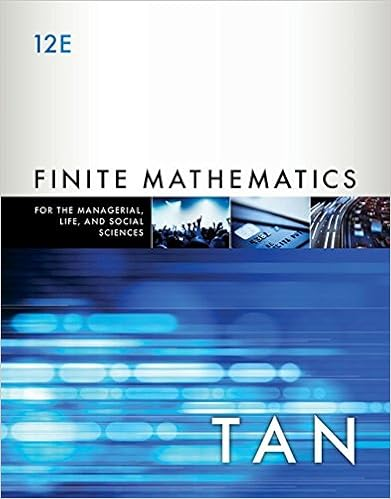# Problem 1 10 pts Let T : V ! V and S : V ! V be two linear...

• Test Prep
• 14

This preview shows page 1 - 6 out of 14 pages.

##### We have textbook solutions for you!
The document you are viewing contains questions related to this textbook.The document you are viewing contains questions related to this textbook.
Chapter 7 / Exercise 64
Finite Mathematics for the Managerial, Life, and Social Sciences: An Applied Approach
TanExpert Verified
Problem 1 (10 pts) Let T : V ! V and S : V ! V be two linear transformations. Show that ST is invertible if and only if S and T are invertible. 2
##### We have textbook solutions for you!
The document you are viewing contains questions related to this textbook.The document you are viewing contains questions related to this textbook.
Chapter 7 / Exercise 64
Finite Mathematics for the Managerial, Life, and Social Sciences: An Applied Approach
TanExpert Verified
Problem 2 (10 pts) Let V, h , i be a real inner product space. Let W be a subspace of V . Define W ? to be the space of all x 2 V such that h x, y i = 0 for all y 2 W i.e: W ? = { x 2 V : h x, y i = 0 for all y 2 W } 1. Prove that W ? is again a subspace of V . 2. Prove that W ? \ W = { 0 } . 3
3. Let x 1 , ..., x k be an orthonormal basis of W . Define T : V ! V by T ( x ) = k i =1 h x, x i i x i . Then T is a linear operator (you may use this without proving it). Prove that the range of T is W and the kernel of T is W ? . 4
4. Prove that dim ( W ) + dim ( W ? ) = dim ( V ) . 5
Problem 3 (10 pts) A matrix U in M n n ( R ) is said to be unitary if U t U = I n 1. Assume that U is unitary and λ is an eigenvalue of U . Prove that λ is 1 or - 1. 2. Give an example of a unitary matrix in M 2 2 ( R ) which does not have a real eigenvalue. 6
•••